Next: Hard-Sphere Scattering Up: Scattering Theory Previous: Optical Theorem

# Determination of Phase-Shifts

Let us now consider how the partial wave phase-shifts,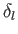, can be evaluated. Consider a spherically symmetric potential,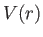, that vanishes for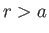, where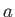is termed the range of the potential. In the region, the wavefunction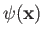satisfies the free-space Schrödinger equation, (10.56). According to Equations (10.70), (10.73), (10.74), and (10.78), the most general solution of this equation that is consistent with no incoming spherical waves, other than those contained in the incident wave, is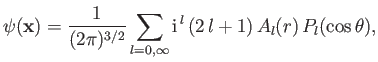(10.93)

where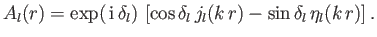(10.94)

Note that Neumann functions are allowed to appear in the previous expression, because its region of validity does not include the origin (where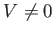). The logarithmic derivative of theth radial wavefunction,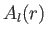, just outside the range of the potential is given by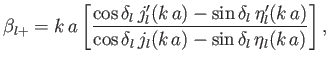(10.95)

where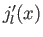denotes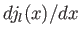, et cetera. The previous equation can be inverted to give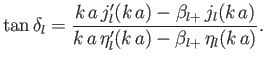(10.96)

Thus, the problem of determining the phase-shift,, is equivalent to that of determining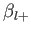.

The most general solution to Schrödinger's equation inside the range of the potential (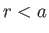) that does not depend on the azimuthal angle,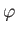, is(10.97)

where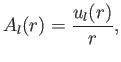(10.98)

and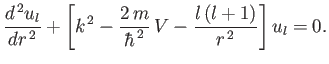(10.99)

(See Exercise 12.) The boundary condition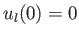(10.100)

ensures that the radial wavefunction is well behaved at the origin. We can launch a well-behaved solution of the previous equation from, integrate out to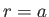, and form the logarithmic derivative [of]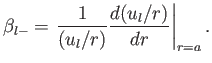(10.101)

Becauseand its first derivatives are necessarily continuous for physically acceptable wavefunctions, it follows that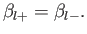(10.102)

The phase-shift,, is then obtained from Equation (10.97).Next: Hard-Sphere Scattering Up: Scattering Theory Previous: Optical Theorem
Richard Fitzpatrick 2016-01-22